# 一堂精彩绝伦的算法课

## 前情提要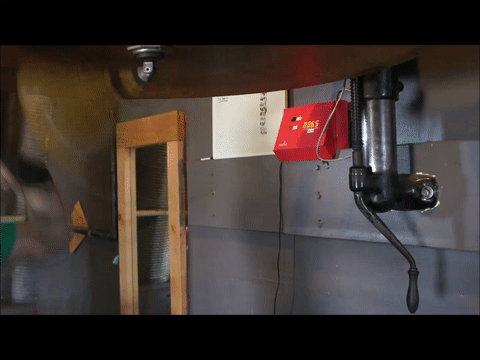``````/*
BeatBag - A Speed Bag Counter打击沙袋-一个击打次数统计器
Nathan Seidle（老板名字）
SparkFun Electronics火花快乐电子
2/23/2013（代码编写时间）

License: This code is public domain but you buy me a beer if you use this and we meet someday (Beerware license).

BeatBag is a speed bag counter that uses an accelerometer to counts the number hits. 打击沙袋是一个用加速计来统计沙袋被击打次数的装置。
It's easily installed ontop of speed bag platform only needing an accelerometer attached to the top of platform. 它很容易安装在速度袋平台的顶部，只需要一个加速度计连接到平台的顶部。
You don't have to alter the hitting surface or change out the swivel.
你不需改变击球表面或改装它。

I combine X/Y/Z into one vector and look only at the magnitude.

I use a fourth order filter to see the impacts (accelerometer peaks) from the speed bag. It works pretty well.

It's very reproducible but I'm not entirely sure how accurate it is. I can detect both bag hits (forward/backward) then I divide by two to get the number displayed to the user.

I arrived at the peak detection algorithm using video and raw data recordings. After a fourth filtering I could glean the peaks. There is probably a much better way to do the math on the peak detection but it's not one of my strength.

Hardware setup:硬件连线指导：
5V from wall supply goes into barrel jack on Redboard. Trace cut to diode. RedBoard barel jack is wired to power switch then to Vin diode. Display gets power from Vin and data from I2C pins Vcc/Gnd from RedBoard goes into Bread Board Power supply that supplies 3.3V to accelerometer. Future versions should get power from 3.3V rail on RedBoard.

MMA8452 Breakout ------------ Arduino
3.3V --------------------- 3.3V
SDA(yellow) -------^^(330)^^------- A4
SCL(blue) -------^^(330)^^------- A5
GND ---------------------- GND
The MMA8452 is 3.3V so we recommend using 330 or 1k resistors between a 5V Arduino and the MMA8452 breakout.
The MMA8452 has built in pull-up resistors for I2C so you do not need additional pull-ups.

3/2/2013 - Got data from Hugo and myself, 3 rounds, on 2g setting. Very noisy but mostly worked

12/19/15 - Segment burned out. Power down display after 10 minutes of non-use.
Use I2C, see if we can avoid the 'multiply by 10' display problem.

1/23/16 - Accel not reliable. Because the display is now also on the I2C the pull-up resistors on the accel where not enough. Swapped out to new accel. Added 100 ohm inline resistors to accel and 4.7k resistors from SDA/SCL to 5V.
Reinforced connection from accel to RedBoard.

*/

#include <avr/wdt.h> //We need watch dog for this program

#include <Wire.h> // Used for I2C

int hitCounter = 0; //Keeps track of the number of hits

const int resetButton = 6; //Button that resets the display and counter
const int LED = 13; //Status LED on D3

long lastPrint; //Used for printing updates every second

boolean displayOn; //Used to track if display is turned off or not

//Used in the new algorithm
float lastMagnitude = 0;
float lastFirstPass = 0;
float lastSecondPass = 0;
float lastThirdPass = 0;
long lastHitTime = 0;
int secondsCounter = 0;

//This was found using a spreadsheet to view raw data and filter it
const float WEIGHT = 0.9;

//This was found using a spreadsheet to view raw data and filter it
const int MIN_MAGNITUDE_THRESHOLD = 1000; //350 is good

//This is the minimum number of ms between possible hits
//We use this to filter out peaks that are too close together
const int MIN_TIME_BETWEEN_HITS = 90; //100 works well

//This is the number of miliseconds before we turn off the display
long TIME_TO_DISPLAY_OFF = 60L * 1000L * 5L; //5 minutes of no use

int DEFAULT_BRIGHTNESS = 50; //50% brightness to avoid burning out segments after 3 years of use

unsigned long currentTime; //Used for millis checking

void setup()
{
wdt_reset(); //Pet the dog
wdt_disable(); //We don't want the watchdog during init

pinMode(resetButton, INPUT_PULLUP);
pinMode(LED, OUTPUT);

//By default .begin() will set I2C SCL to Standard Speed mode of 100kHz
Wire.setClock(400000); //Optional - set I2C SCL to High Speed Mode of 400kHz
Wire.begin(); //Join the bus as a master

Serial.begin(115200);
Serial.println("Speed Bag Counter");

initDisplay();

clearDisplay();
Wire.print("Accl"); //Display an error until accel comes online
Wire.endTransmission();

while(!initMMA8452()) //Test and intialize the MMA8452
; //Do nothing

clearDisplay();
Wire.print("0000");
Wire.endTransmission();

lastPrint = millis();
lastHitTime = millis();

wdt_enable(WDTO_250MS); //Unleash the beast
}

void loop()
{
wdt_reset(); //Pet the dog

currentTime = millis();
if ((unsigned long)(currentTime - lastPrint) >= 1000)
{
digitalWrite(LED, HIGH);
else
digitalWrite(LED, LOW);

lastPrint = millis();
}

//See if we should power down the display due to inactivity
if (displayOn == true)
{
currentTime = millis();
if ((unsigned long)(currentTime - lastHitTime) >= TIME_TO_DISPLAY_OFF)
{
Serial.println("Power save");

hitCounter = 0; //Reset the count

clearDisplay(); //Clear to save power
displayOn = false;
}
}

//Check the accelerometer
float currentMagnitude = getAccelData();

//Send this value through four (yes four) high pass filters
float firstPass = currentMagnitude - (lastMagnitude * WEIGHT) - (currentMagnitude * (1 - WEIGHT));
lastMagnitude = currentMagnitude; //Remember this for next time around

float secondPass = firstPass - (lastFirstPass * WEIGHT) - (firstPass * (1 - WEIGHT));
lastFirstPass = firstPass; //Remember this for next time around

float thirdPass = secondPass - (lastSecondPass * WEIGHT) - (secondPass * (1 - WEIGHT));
lastSecondPass = secondPass; //Remember this for next time around

float fourthPass = thirdPass - (lastThirdPass * WEIGHT) - (thirdPass * (1 - WEIGHT));
lastThirdPass = thirdPass; //Remember this for next time around
//End high pass filtering

fourthPass = abs(fourthPass); //Get the absolute value of this heavily filtered value

//See if this magnitude is large enough to care
if (fourthPass > MIN_MAGNITUDE_THRESHOLD)
{
//We have a potential hit!

currentTime = millis();
if ((unsigned long)(currentTime - lastHitTime) >= MIN_TIME_BETWEEN_HITS)
{
//We really do have a hit!
hitCounter++;

lastHitTime = millis();

//Serial.print("Hit: ");
//Serial.println(hitCounter);

if (displayOn == false) displayOn = true;

}
}

//Check if we need to reset the counter and display
{
//This breaks the file up so we can see where we hit the reset button
Serial.println();
Serial.println();
Serial.println("Reset!");
Serial.println();
Serial.println();

hitCounter = 0;

resetDisplay(); //Forces cursor to beginning of display

while (digitalRead(resetButton) == LOW) wdt_reset(); //Pet the dog while we wait for you to remove finger

//Do nothing for 250ms after you press the button, a sort of debounce
for (int x = 0 ; x < 25 ; x++)
{
wdt_reset(); //Pet the dog
delay(10);
}
}
}

//This function makes sure the display is at 57600
void initDisplay()
{
resetDisplay(); //Forces cursor to beginning of display

printHits(); //Update display with current hit count

displayOn = true;

setBrightness(DEFAULT_BRIGHTNESS);
}

//Set brightness of display
void setBrightness(int brightness)
{
Wire.write(0x7A); // Brightness control command
Wire.write(brightness); // Set brightness level: 0% to 100%
Wire.endTransmission();
}

void resetDisplay()
{
//Send the reset command to the display - this forces the cursor to return to the beginning of the display
Wire.write('v');
Wire.endTransmission();

if (displayOn == false)
{
setBrightness(DEFAULT_BRIGHTNESS); //Power up display
displayOn = true;
lastHitTime = millis();
}
}

//Push the current hit counter to the display
void printHits()
{
int tempCounter = hitCounter / 2; //Cut in half

Wire.write(0x79); //Move cursor
Wire.write(4); //To right most position

Wire.write(tempCounter / 1000); //Send the left most digit
tempCounter %= 1000; //Now remove the left most digit from the number we want to display
Wire.write(tempCounter / 100);
tempCounter %= 100;
Wire.write(tempCounter / 10);
tempCounter %= 10;
Wire.write(tempCounter); //Send the right most digit

Wire.endTransmission(); //Stop I2C transmission
}

//Clear display to save power (a screen saver of sorts)
void clearDisplay()
{
Wire.write(0x79); //Move cursor
Wire.write(4); //To right most position

Wire.write(' ');
Wire.write(' ');
Wire.write(' ');
Wire.write(' ');

Wire.endTransmission(); //Stop I2C transmission
}
``````

1. 关于算法的专业造诣和技能，我真的知之甚少。
2. 我的这些近似方法非常的不准确。平均误差竟有28%。我认为这是因为当拳击手进入比较固定的出击节奏时，有一些谐波的频率会破坏。

1. 写一个可以获得正确击打次数的算法并包含相关的数据输出。(数据从这里获取).
2. 将此算法运用到某种微控制器上，8位或32位的都行，别上FPGA，PLC之类的，除非你疯了。
3. 最重要的是：写下你解决这个问题的一系列详细步骤，你如何对数据进行滤波并最终解决问题，我们在这里等着向你学习。
4. 将你的代码和文档发到我们相关的反馈途径或者公布到网上。
5. 用你的算法来告诉我们未知数据1未知数据2到底有多少次击打。当比赛结束后，我们会公布这两个过程的视频来求证算法的可靠程度。

## 从何处开始Where to Start

• Requirements需求分析
• Design架构设计
• Implementation代码实施
• Test结果测试

• 该算法需要能够从原始记录的数据中获得正确的击打次数。
• 该算法能够在8位或者32位的微控制器上实现
• 撰写文档和教程帮助大家了解解决问题过程中的思路和方法
• 将代码和文档在网站或者公共托管的地方发布
• 测试你的算法用于数据记录的结果，并用于未知的数据记录并给出结果。
• 加速度计放置在沙袋上方的基座，Z轴正向朝上方，负向朝下方。
• 数据记录需要更加本质的处理算法，你需要根据来源的振幅校正已有数据-正如Nate所猜测的那样，共振可能是问题的源头。
• 你有15天的时间完成此项目（哇哈！）

## 创建建解决方案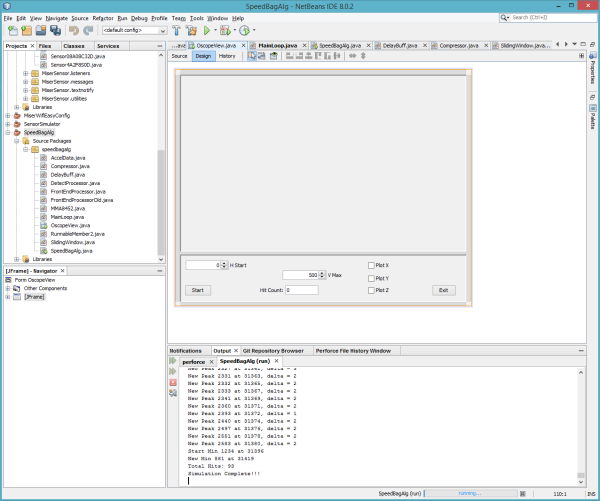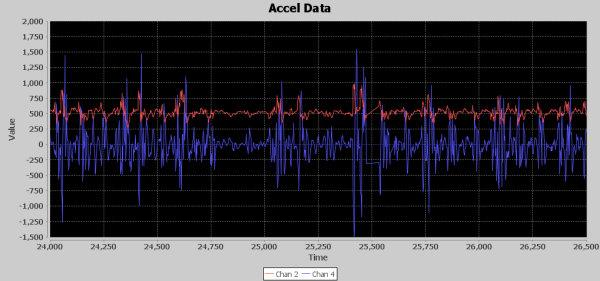## 循序渐进，摸着石头过河，小步迭代验证方案

- 美国海军上将迈耶斯

### runF1

runF1() 仅使用Z轴，并且使用Z数据的均值去掉偏置，使用滑动窗口和滤波。 我创建了一个称为延迟的元素，这是一种延迟输入数据的方式，因此可以稍后与平均结果的输出对齐。 这允许基于周围值而不是先前的值从Z轴数据中减去滑动窗口平均值。 击打检测使用大于五个样本的平均值的放大滤波器数据的直接比较，在检测之间的最短时差为250毫秒。

### runF2

runF2() used only Z axis, and employed weak bias removal via a sliding window but added dynamic beta amplification of the filtered Z data based on the average amplitude above the bias that was removed when the last punch was detected. Also, a dynamic minimum time between punches of 225ms to 270ms was calculated based on delta time since last punch was detected. I called the amount of bias removed noise floor. I added a button to stop and resume the simulation so I could examine the debug output and the waveforms. This allowed me to see the beta amplification being used as the simulation went along.

### runF3

runF3() used X and Z axis data. My theory was that there might be a jolt of movement from the punching action that could be additive to the Z axis data to help pinpoint the actual punch. It was basically the same algorithm as RunF2 but added in the X axis. It actually worked pretty well, and I thought I might be onto something here by correlating X movement and Z. I tried various tweaks and gyrations as you can see in the code lots of commented out experiments. I started playing around with what I call a compressor, which took the sum of five samples to see if it would detect bunches of energy around when punches occur. I didn’t use it in the algorithm but printed out how many times it crossed a threshold to see if it had any potential as a filtering element. In the end, this algorithm started to implode on itself, and it was time to take what I learned and start a new algorithm.

### runF4

In runF4(), I increased the bias removal average to 50 samples. It started to work in attenuation and sample compression along with a fixed point LSB to preserve some decimal precision to the integer attenuate data. Since one of the requirements was this should be able to run on 8-bit microcontrollers, I wanted to avoid using floating point and time consuming math functions in the final C/C++ code. I’ll speak more to this in the components section, but, for now, know that I’m starting to work this in. I’ve convinced myself that finding bursts of acceleration is the way to go. At this point, I am removing the bias from both Z and X axis then squaring. I then attenuate each, adding the results together but scaling X axis value by 10. I added a second stage of averaging 11 filtered values to start smoothing the bursts of acceleration. Next, when the smoothed value gets above a fixed threshold of 100, the unsmoothed combination of Z and X squared starts getting loaded into the compressor until 100 samples have been added. If the compressor output of the 100 samples is greater than 5000, it is recorded as a hit. A variable time between punches gate is employed, but it is much smaller since the compressor is using 100 samples to encapsulate the punch detection. This lowers the gate time to between 125 and 275 milliseconds. While showing some promise, it was still too sensitive. While one data set would be spot on another would be off by 10 or more punches. After many tweaks and experiments, this algorithm began to implode on itself, and it was once again time to take what I’ve learned and start anew. I should mention that at this tim I’m starting to think there might not be a satisfactory solution to this problem. The resonant vibrations that seem to be out of phase with the contacts of the bag just seems to wreak havoc on the acceleration seen when the boxer gets into a good rhythm. Could this all just be a waste of time?

### runF5

runF5()’s algorithm started out with the notion that a more formal high pass filter needed to be introduced rather than an average subtracted from the signal. The basic premise of the high pass filter was to use 99% of the value of new samples added to 1% of the value of average. An important concept added towards the end of runF5’s evolution was to try to simplify the algorithm by removing the first stage of processing into its own file to isolate it from later stages. Divide and Conquer; it’s been around forever, and it really holds true time and time again. I tried many experiments as you can see from the many commented out lines in the algorithm and in the FrontEndProcessorOld.java file. In the end, it was time to carry forward the new Front End Processor concept and start anew with divide and conquer and a need for a more formal high pass filter.

### runF6

With time running out, it’s time to pull together all that has been learned up to now, get the Java code ready to port to C/C++ and implement real filters as opposed to using running averages. In runF6(), I had been pulling together the theory that I need to filter out the bias on the front end with a high pass filter and then try to use a low pass filter on the remaining signal to find bursts of acceleration that occur at a 2 to 4 Hertz frequency. No way was I going to learn how to calculate my own filter tap values to implement the high and low pass filters in the small amount of time left before the deadline. Luckily, I discovered the t-filter web site. Talk about a triple play. Not only was I able to put in my parameters and get filter tap values, I was also able to leverage the C code it generated with a few tweaks in my Java code. Plus, it converted the tap values to fixed point for me! Fully employing the divide and conquer concept, this final version of the algorithm introduced isolated sub algorithms for both Front End Processor and Detection Processing. This allowed me to isolate the two functions from each other except for the output signal of one becoming the input to the other, which enabled me to focus easily on the task at hand rather than sift through a large group of variables where some might be shared between the two stages.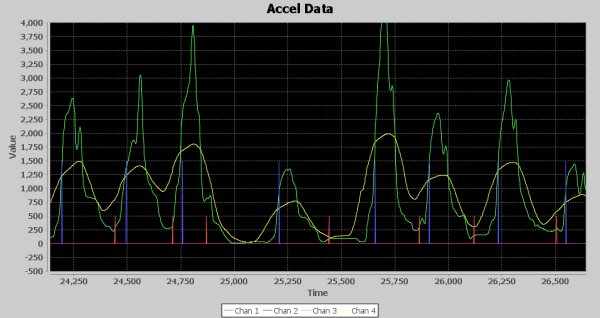Final output of DET

Above is the visual output from runF6. The Green line is 45 samples delayed of the output of the low pass filter, and the yellow line is an average of 99 values of the output of the low pass filter. The Detection Processor includes a detection algorithm that detects punches by tracking min and max crossings of the Green signal using the Yellow signal as a template for dynamic thresholding. Each minimum is a Red spike, and each maximum is a Blue spike, which is also a punch detection. The timescale is in milliseconds. Notice there are about three blue spikes per second inside the 2 to 4Hz range predicted. And the rest is history!

## 算法构成

### Delay

This is used to buffer a signal so you can time align it to some other operation. For example, if you average nine samples and you want to subtract the average from the original signal, you can use a delay of five samples of the original signal so you can use values that are itself plus the four samples before and four samples after.

### Attenuate

Attenuation is a simple but useful operation that can scale a signal down before it is amplified in some fashion with filtering or some other operation that adds gain to the signal. Typically attenuation is measured in decibels (dB). You can attenuate power or amplitude depending on your application. If you cut the amplitude by half, you are reducing it by -6 dB. If you want to attenuate by other dB values, you can check the dB scale here. As it relates to the Speedbag algorithm, I’m basically trying to create clear gaps in the signal, for instance squelching or squishing smaller values closer to zero so that squaring values later can really push the peaks higher but not having as much effect on the values pushed down towards zero. I used this technique to help accentuate the bursts of acceleration versus background vibrations of the speed bag platform.

### Sliding Window Average

Sliding Window Average is a technique of calculating a continuous average of the incoming signal over a given window of samples. The number of samples to be averaged is known as the window size. The way I like to implement a sliding window is to keep a running total of the samples and a ring buffer to keep track of the values. Once the ring buffer is full, the oldest value is removed and replaced with the next incoming value, and the value removed from the ring buffer is subtracted from the new value. That result is added to the running tally. Then simply divide the running total by the window size to get the current average whenever needed.

### Rectify

This is a very simple concept which is to change the sign of the values to all positive or all negative so they are additive. In this case, I used rectification to change all values to positive. As with rectification, you can use a full wave or half wave method. You can easily do full wave by using the `abs()` math function that returns the value as positive. You can square values to turn them positive, but you are changing the amplitude. A simple rectify can turn them positive without any other effects. To perform half wave rectification, you can just set any value less than zero to zero.

### Compression

In the DSP world Compression is typically defined as compressing the amplitudes to keep them in a close range. My compression technique here is to sum up the values in a window of samples. This is a form of down-sampling as you only get one sample out each time the window is filled, but no values are being thrown away. It’s a pure total of the window, or optionally an average of the window. This was employed in a few of the algorithms to try to identify bursts of acceleration from quieter times. I didn’t actually use it in the final algorithm.

### FIR Filter

Finite Impulse Response (FIR) is a digital filter that is implemented via a number of taps, each with its assigned polynomial coefficient. The number of taps is known as the filter’s order. One strength of the FIR is that it does not use any feedback, so any rounding errors are not cumulative and will not grow larger over time. A finite impulse response simply means that if you input a stream of samples that consisted of a one followed by all zeros, the output of the filter would go to zero within at most the order +1 amount of 0 value samples being fed in. So, the response to that single sample of one lives for a finite amount of samples and is gone. This is essentially achieved by the fact there isn’t any feedback employed. I’ve seen DSP articles claim calculating filter tap size and coefficients is simple, but not to me. I ended up finding an online app called tFilter that saved me a lot of time and aggravation. You pick the type of filter (low, high, bandpass, bandstop, etc) and then setup your frequency ranges and sampling frequency of your input data. You can even pick your coefficients to be produced in fixed point to avoid using floating point math. If you’re not sure how to use fixed point or never heard of it, I’ll talk about that in the Embedded Optimization Techniques section.

## 基于嵌入式目标运行机进行优化

### Magnitude Squared

Mag Square is a technique that can save computing power of calculating square roots. For example, if you want to calculate the vector for X and Z axis, normally you would do the following: val = sqr((X * X) + (Y * Y)). However, you can simply leave the value in (X * X) + (Y * Y), unless you really need the exact vector value, the Mag Square gives you a usable ratio compared to other vectors calculated on subsequent samples. The numbers will be much larger, and you may want to use attenuation to make them smaller to avoid overflow from additional computation downstream.

I used this technique in the final algorithm to help accentuate the bursts of acceleration from the background vibrations. I only used Z * Z in my calculation, but I then attenuated all the values by half or -6dB to bring them back down to reasonable levels for further processing. For example, after removing the bias if I had some samples around 2 and then some around 10, when I squared those values I now have 4 and 100, a 25 to 1 ratio. Now, if I attenuate by .5, I have 2 and 50, still a 25 to 1 ratio but now with smaller numbers to work with.

### Fixed Point

Using fixed point numbers is another way to stretch performance, especially on microcontrollers. Fixed point is basically integer math, but it can keep precision via an implied fixed decimal point at a particular bit position in all integers. In the case of my FIR filter, I instructed tFilter to generate polynomial values in 16-bit fixed point values. My motivation for this was to ensure I don’t use more than 32-bit integers, which would especially hurt performance on an 8-bit microcontroller.

Rather than go into the FIR filter code to explain how fixed point works, let me first use a simple example. While the FIR filter algorithm does complex filtering with many polynomials, we could implement a simple filter that outputs the same input signal but -6dB down or half its amplitude. In floating point terms, this would be a simple one tap filter to multiply each incoming sample by 0.5. To do this in fixed point with 16 bit precision, we would need to convert 0.5 into its 16-bit fixed point representation. A value of 1.0 is represented by 1 * (216) or 65,536. Anything less than 65536 is a value less than 1. To create a fixed point integer of 0.5, we simply use the same formula 0.5 * (216), which equals 32,768. Now we can use that value to lower the amplitude by .5 of every sample input. For example, say we input into our simple filter a sample with the value of 10. The filter would calculate 10 * 32768 = 327,680, which is the fixed point representation. If we no longer care about preserving the precision after the calculations are performed, it can easily be turned back into a non-fixed point integer by simply right shifting by the number of bits of precision being used. Thus, 327680 >> 16 = 5. As you can see, our filter changed 10 into 5 which of course is the one half or -6dB we wanted out. I know 0.5 was pretty simple, but if you had wanted 1/8 the amplitude, the same process would be used, 65536 * .125 = 8192. If we input a sample of 16, then 16 * 8192 = 131072, now change it back to an integer 131072 >> 16 = 2. Just to demonstrate how you lose the precision when turning back to integer (the same as going float to integer) if we input 10 into the 1/8th filter it would yield the following, 10 * 8192 = 81920 and then turning it back to integer would be 81920 >> 16 = 1, notice it was 1.25 in fixed point representation.

Getting back to the FIR filters, I picked 16 bits of precision, so I could have a fair amount of precision but balanced with a reasonable amount of whole numbers. Normally, a signed 32-bit integer can have a range of - 2,147,483,648 to +2,147,483,647, however there now are only 16 bits of whole numbers allowed which is a range of -32,768 to +32,767. Since you are now limited in the range of numbers you can use, you need to be cognizant of the values being fed in. If you look at the FEPFilter_get function, you will see there is an accumulator variable accZ which sums the values from each of the taps. Usually if your tap history values are 32 bit, you make your accumulator 64-bit to be sure you can hold the sum of all tap values. However, you can use a 32 bit value if you ensure that your input values are all less than some maximum. One way to calculate your maximum input value is to sum up the absolute values of the coefficients and divide by the maximum integer portion of the fixed point scheme. In the case of the FEP FIR filter, the sum of coefficients was 131646, so if the numbers can be 15 bits of positive whole numbers + 16 bits of fractional numbers, I can use the formula (231)/131646 which gives the FEP maximum input value of + or - 16,312. In this case, another optimization can be realized which is not to have a microcontroller do 64-bit calculations.

## Walking the Signal Processing Chain

### Delays Due to Filtering

Before walking through the processing chain, we should discuss delays caused by filtering. Many types of filtering add delays to the signal being processed. If you do a lot of filtering work, you are probably well aware of this fact, but, if you are not all that experienced with filtering signals, it’s something of which you should be aware. What do I mean by delay? This simply means that if I put in a value X and I get out a value Y, how long it takes for the most impact of X to show up in Y is the delay. In the case of a FIR filter, it can be easily seen by the filter’s Impulse response plot, which, if you remember from my description of FIR filters, is a stream of 0’s with a single 1 inserted. T-Filter shows the impulse response, so you can see how X impacts Y’s output. Below is an image of the FEP’s high pass filter Impulse Response taken from the T-Filter website. Notice in the image that the maximum impact on X is exactly in the middle, and there is a point for each tap in the filter.Below is a diagram of a few of the FEP’s high pass filter signals. The red signal is the input from the accelerometer or the newest sample going into the filter, the blue signal is the oldest sample in the filter’s ring buffer. There are 19 taps in the FIR filter so they represent a plot of the first and last samples in the filter window. The green signal is the value coming out of the high pass filter. So to relate to my X and Y analogy above, the red signal is X and the green signal is Y. The blue signal is delayed by 36 milliseconds in relation to the red input signal which is exactly 18 samples at 2 milliseconds, this is the window of data that the filter works on and is the Finite amount of time X affects Y.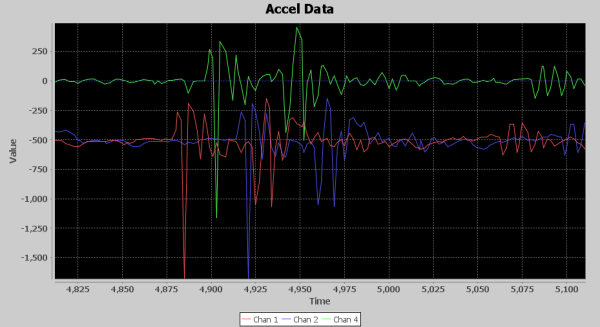Notice the output of the high pass filter (green signal) seems to track changes from the input at a delay of 18 milliseconds, which is 9 samples at 2 milliseconds each. So, the most impact from the input signal is seen in the middle of the filter window, which also coincides with the Impulse Response plot where the strongest effects of the 1 value input are seen at the center of the filter window.

It’s not only a FIR that adds delay. Usually, any filtering that is done on a window of samples will cause a delay, and, typically, it will be half the window length. Depending on your application, this delay may or may not have to be accounted for in your design. However, if you want to line this signal up with another unfiltered or less filtered signal, you are going to have to account for it and align it with the use of a delay component.

### Front End Processor

I’ve talked at length about how to get to a final solution and all the components that made up the solution, so now let’s walk through the processing chain and see how the signal is transformed into one that reveals the punches. The FEP’s main goal is to remove bias and create an output signal that smears across the bursts of acceleration to create a wave that is higher in amplitude during increased acceleration and lower amplitude during times of less acceleration. There are four serial components to the FEP: a High Pass FIR, Attenuator, Rectifier and Smoothing via Sliding Window Average.

The first image is the input and output of the High Pass FIR. Since they are offset by the amount of bias, they don’t overlay very much. The red signal is the input from the accelerometer, and the blue is the output from the FIR. Notice the 1g of acceleration due to gravity is removed and slower changes in the signal are filtered out. If you look between 24,750 and 25,000 milliseconds, you can see the blue signal is more like a straight line with spikes and a slight ringing on it, while the original input has those spikes but meandering on some slow ripple.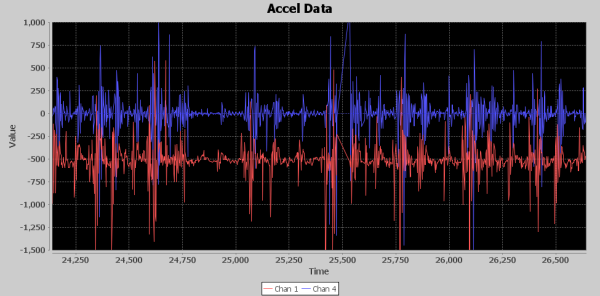Next is the output of the attenuator. While this component works on the entire signal, it lowers the peak values of the signal, but its most important job is to squish the quieter parts of the signal closer to zero values. The image below shows the output of the attenuator, and the input was the output of the High Pass FIR. As expected, peaks are much lower but so is the quieter time. This makes it a little easier to see the acceleration bursts.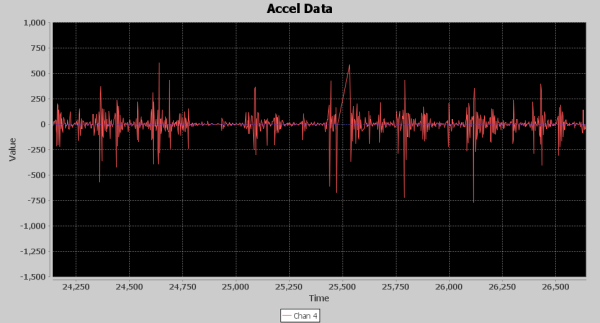Next is the rectifier component. Its job is to turn all the acceleration energy in the positive direction so that it can be used in averaging. For example, an acceleration causing a positive spike of 1000 followed by a negative spike of 990 would yield an average of 5, while a 1000 followed by a positive of 990 would yield an average of 995, a huge difference. Below is an image of the Rectifier output. The bursts of acceleration are slightly more visually apparent, but not easily discernable. In fact, this image shows exactly why this problem is such a tough one to solve; you can clearly see how resonant shaking of the base causes the pattern to change during punch energy being added. The left side is lower and more frequent peaks, the right side has higher but less frequent peaks.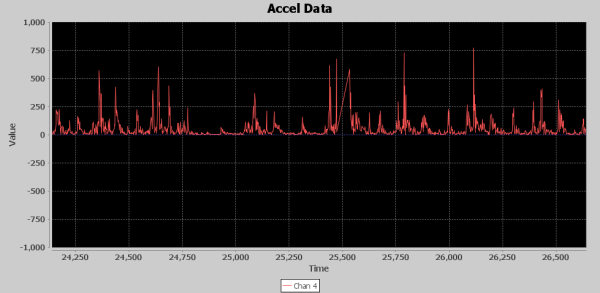The 49 value sliding window is the final step in the FEP. While we have done subtle changes to the signal that haven’t exactly made the punches jump out in the images, this final stage makes it visually apparent that the signal is well on its way of yielding the hidden punch information. The fruits of the previous signal processing magically show up at this stage. Below is an image of the Sliding Window average. The blue signal is its input or the output of the Rectifier, and the red signal is the output of the sliding window. The red signal is also the final output of the FEP stage of processing. Since it is a window, it has a delay associated with it. Its approximately 22 samples or 44 milliseconds on average. It doesn’t always look that way because sometimes the input signal spikes are suddenly tall with smaller ringing afterwards. Other times there are some small spikes leading up to the tall spikes and that makes the sliding window average output appear inconsistent in its delay based on where the peak of the output shows up. Although these bumps are small, they are now representing where new acceleration energy is being introduced due to punches.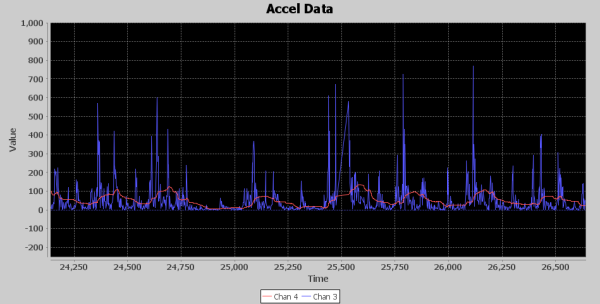### Detection Processor

Now it’s time to move on to the Detection Processor (DET). The FEP outputs a signal that is starting to show where the bursts of acceleration are occurring. The DET’s job will be to enhance this signal and employ an algorithm to detect where the punches are occurring.

The first stage of the DET is an attenuator. Eventually, I want to add exponential gain to the signal to really pull up the peaks, but, before doing that, it is important to once again squish down the lower values towards zero and lower the peaks to keep from generating values too large to process in the rest of the DET chain. Below is an image of the output from the attenuator stage, it looks just like the signal output from the FEP, however notice the signal level peaks were above 100 from the FEP, and now peaks are barely over 50. The vertical scale is zoomed in with the max amplitude set to 500 so you can see that there is a viable signal with punch information.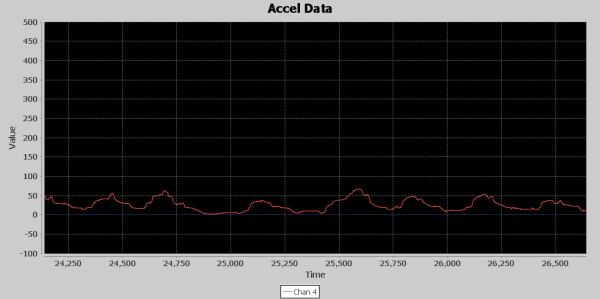With the signal sufficiently attenuated, it’s time to create the magic. The Magnitude Square function is where it all comes together. The attenuated signal carries the tiny seeds from which I’ll grow towering Redwoods. Below is an image of the Mag Square output, the red signal is the attenuated input, and the blue signal is the mag square output. I’ve had to zoom out to a 3,000 max vertical, and, as you can see, the input signal almost looks flat, yet the mag square was able to pull out unmistakable peaks that will aid the detection algorithm to pick out punches. You might ask why not just use these giant peaks to detect punches. One of the reasons I’ve picked this area of the signal to analyze is to show you how the amount of acceleration can vary greatly as you can see the peak between 25,000 and 25,250 is much smaller than the surrounding peaks, which makes pure thresholding a tough chore.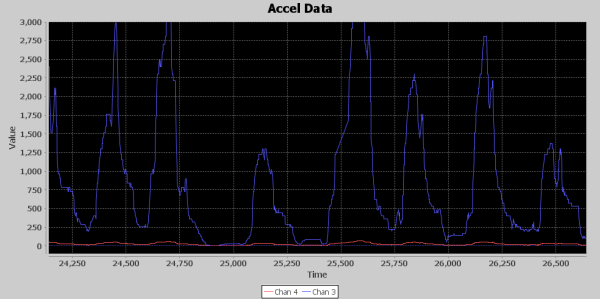Next, I decided to put a Low Pass filter to try to remove any fast changing parts of the signal since I’m looking for events that occur in the 2 to 4 Hz range. It was tough on T-Filter to create a tight low pass filter with a 0 to 5 Hz band pass as it was generating filters with over 100 taps, and I didn’t want to take that processing hit, not to mention I would then need a 64-bit accumulator to hold the sum. I relaxed the band pass with a 0 to 19 Hz range and the band stop at 100 to 250 Hz. Below is an image of the low pass filter output. The blue signal is the input, and the red signal is the delayed output. I used this image because it allows the input and output signal to be seen without interfering with each other. The delay is due to 6 sample delay of the low pass FIR, but I have also introduced a 49 sample delay to this signal so that it is aligned in the center of the 99 sample sliding window average that follows in the processing chain. So it is delayed by a total of 55 samples or 110 milliseconds. In this image, you can see the slight amplification of the slow peaks by their height and how it is smoothed as the faster changing elements are attenuated. Not a lot going on here but the signal is a little cleaner, Earl Muntz might suggest I cut the low pass filter out of the circuit, and it might very well work without it.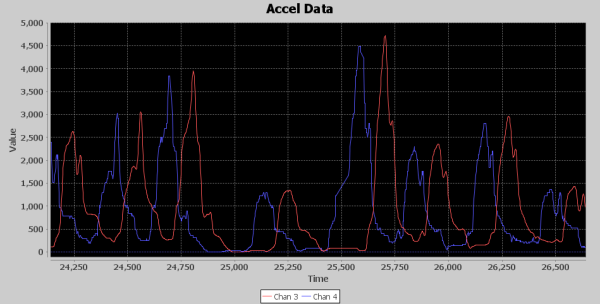The final stage of the signal processing is a 99 sample sliding window average. I built into the sliding window average the ability to return the sample in the middle of the window each time a new value is added and that is how I produced the 49 sample delayed signal in the previous image. This is important because the detection algorithm is going to have 2 parallel signals passed into it, the output of the 99 sliding window average and the 49 sample delayed input into the sliding window average. This will perfectly align the un-averaged signal in the middle of the sliding window average. The averaged signal is used as a dynamic threshold for the detection algorithm to use in its detection processing. Here, once again, is the image of the final output from the DET.In the image, the green and yellow signals are inputs to the detection algorithm, and the blue and red are outputs. As you can see, the green signal, which is a 49 samples delayed, is aligned perfectly with the yellow 99 sliding window average peaks. The detection algorithm monitors the crossing of the yellow by the green signal. This is accomplished by both maximum and minimum start guard state that verifies the signal has moved enough in the minimum or maximum direction in relation to the yellow signal and then switches to a state that monitors the green signal for enough change in direction to declare a maximum or minimum. When the peak start occurs and it’s been at least 260ms since the last detected peak, the state switches to monitor for a new peak in the green signal and also makes the blue spike seen in the image. This is when a punch count is registered. Once a new peak has been detected, the state changes to look for the start of a new minimum. Now, if the green signal falls below the yellow by a delta of 50, the state changes to look for a new minimum of the green signal. Once the green signal minimum is declared, the state changes to start looking for the start of a new peak of the green signal, and a red spike is shown on the image when this occurs.

Again, I’ve picked this time in the recorded data because it shows how the algorithm can track the punches even during big swings in peak amplitude. What’s interesting here is if you look between the 24,750 and 25,000 time frame, you can see the red spike detected a minimum due to the little spike upward of the green signal, which means the state machine started to look for the next start of peak at that point. However, the green signal never crossed the yellow line, so the start of peak state rode the signal all the way down to the floor and waited until the cross of the yellow line just before the 25,250 mark to declare the next start of peak. Additionally, the peak at the 25,250 mark is much lower than the surrounding peaks, but it was still easily detected. Thus, the dynamic thresholding and the state machine logic allows the speed bag punch detector algorithm to “Roll with the Punches”, so to speak.

## 最后感言• 演绎 — 修改、转换或以本作品为基础进行创作
• 在任何用途下，甚至商业目的。
• 只要你遵守许可协议条款，许可人就无法收回你的这些权利。

```https://www.sparkfun.com/news/2115?_ga=1.105187979.946766378.1445226389#requirements

https://learn.sparkfun.com/tutorials/lessons-in-algorithms```